# How to Find Forces

We have previously discussed the procedure for formulate the equation of motion.

https://www.yukimura-physics.com/entry/dyn-f22

The most important part of the procedure is to write down all the forces acting on the object.
If you forget to write them down, or if you write down forces that do not exist, you will not get the correct equation of motion.

However, it is difficult for beginners to find the force without excess or deficiency.
In this lecture, we would like to teach you how to correctly write the forces acting on an object!

## Exercise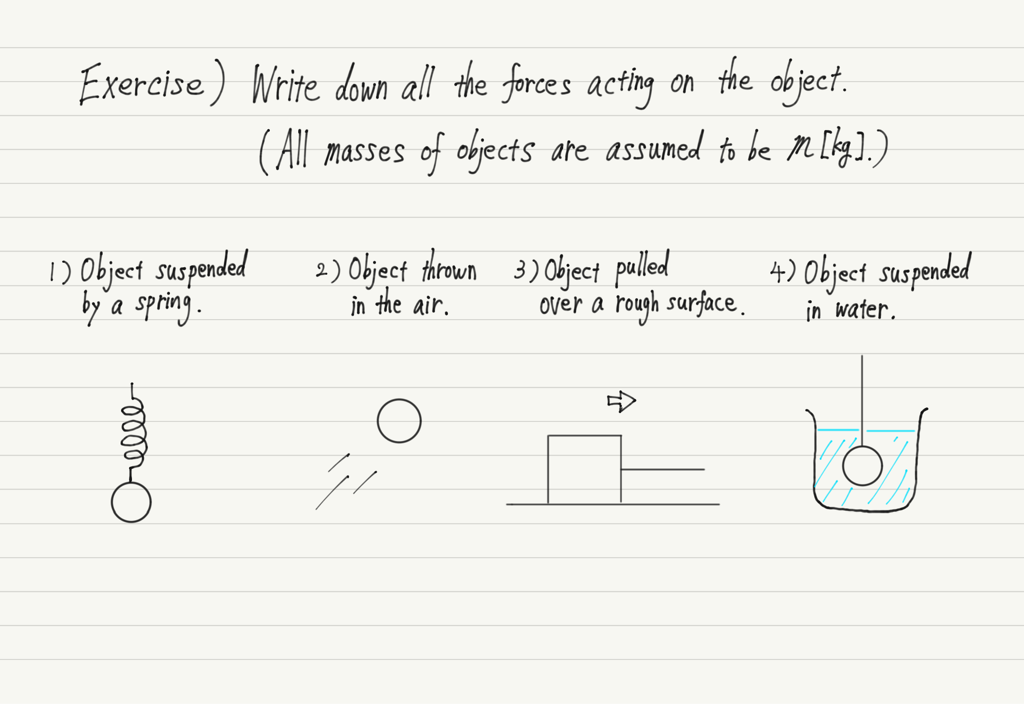I would like to explain this using this example.
The recommended way to find the force is to follow the procedure shown below.

## 1. Write gravitational force

When writing about force, the first thing to do is to write about gravitational force.
Any object is definitely subject to gravitational force as long as it is on the earth.
Therefore, without thinking, let’s write gravitational force first.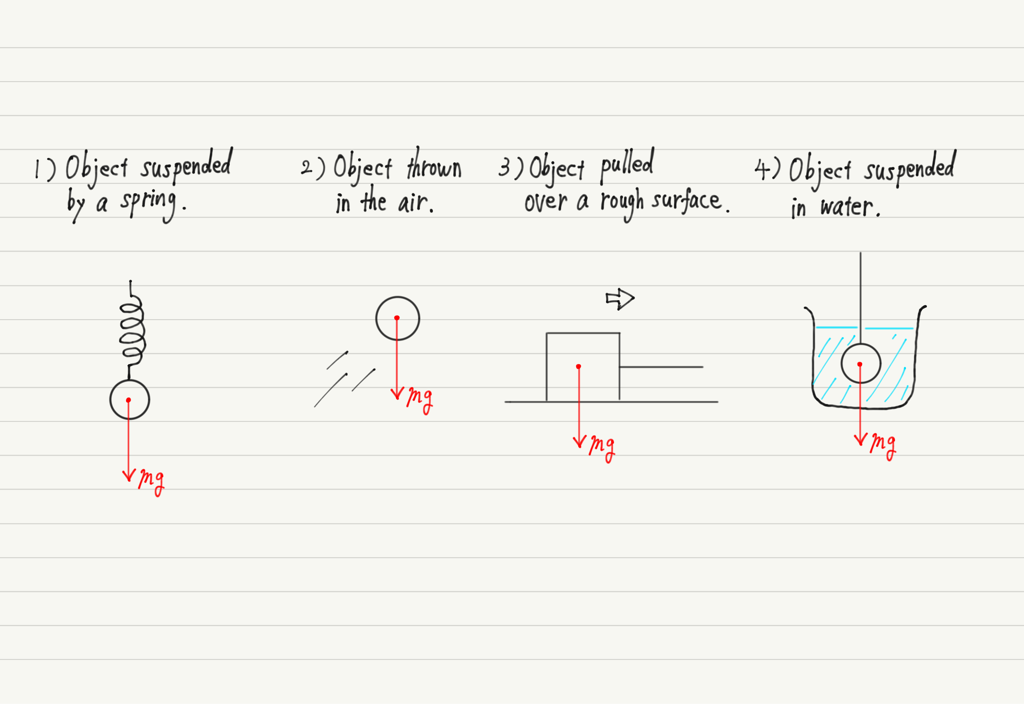## 2. Check for contact with other objects

After writing in the gravitational force, the next step is to focus on the area around the object.

Specifically, check to see if an object is in contact with another object.
An object always receives force from the object it is in contact with.

[Examples]
・Contact with surface → Normal force, Friction
・Contact with string → Tension force
・Contact with spring → Elastic force
・Contact with liquid → Buoyancy
(Air buoyancy and air resistance are often ignored.)

Now, we will write all of these down.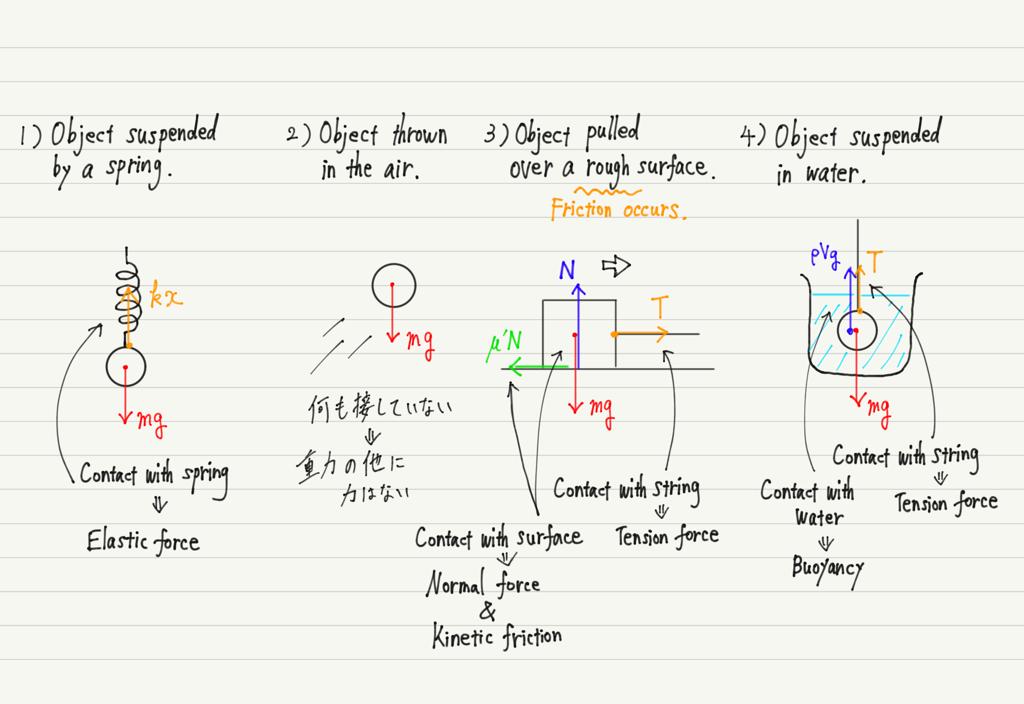Don’t forget to write the magnitude of the force (kx, T, etc.) along with the arrow!

## 3. Don’t write extra force!

Once you have written down gravitational force and the force from the point of contact, there are no other forces acting on the object.
So now it’s complete!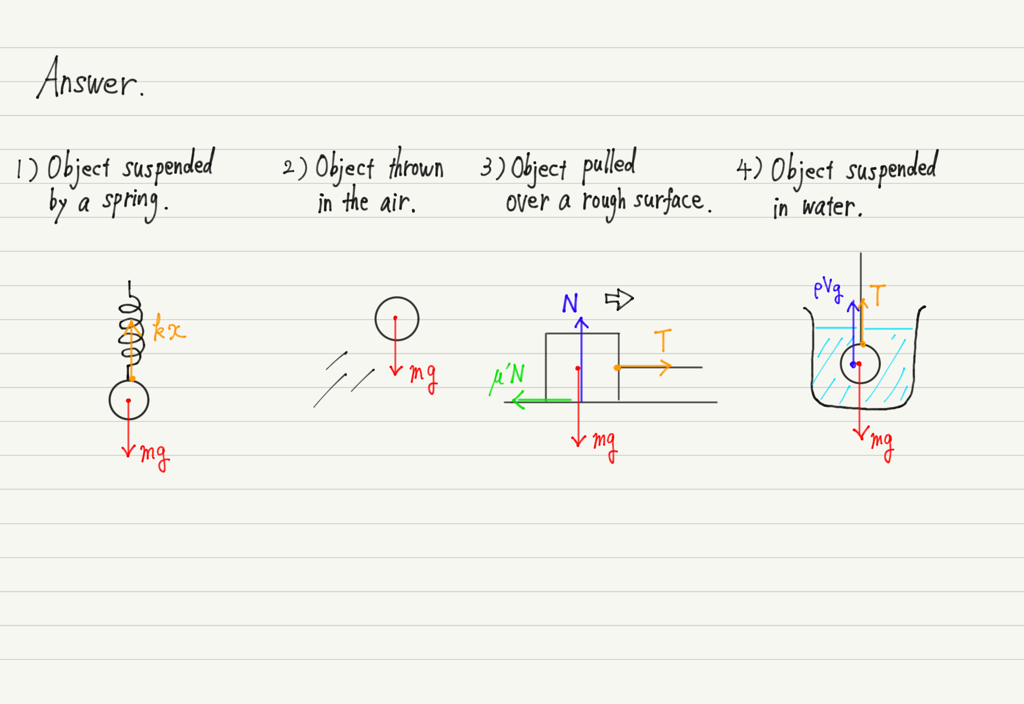You should be able to understand the procedure of force writing and confidently say, “Now it’s complete!”

There is a common mistake made by people who are not confident in their answers.
It is to add a force that does not exist.
In fact, many people make mistake 2).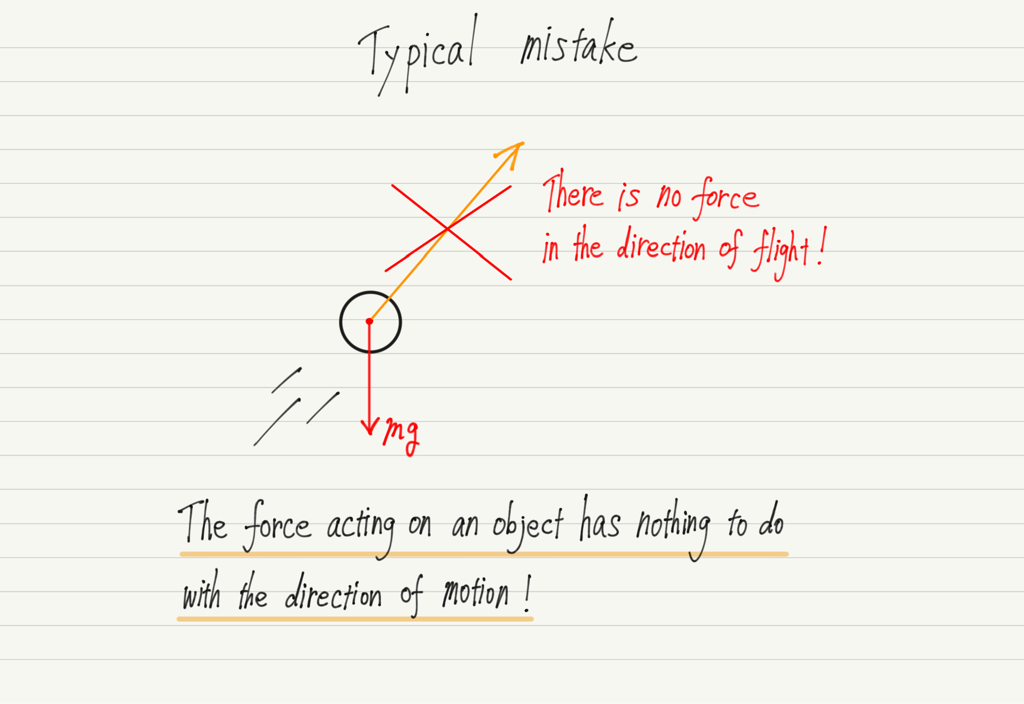## When two objects

Now, let’s finish up with a final exercise.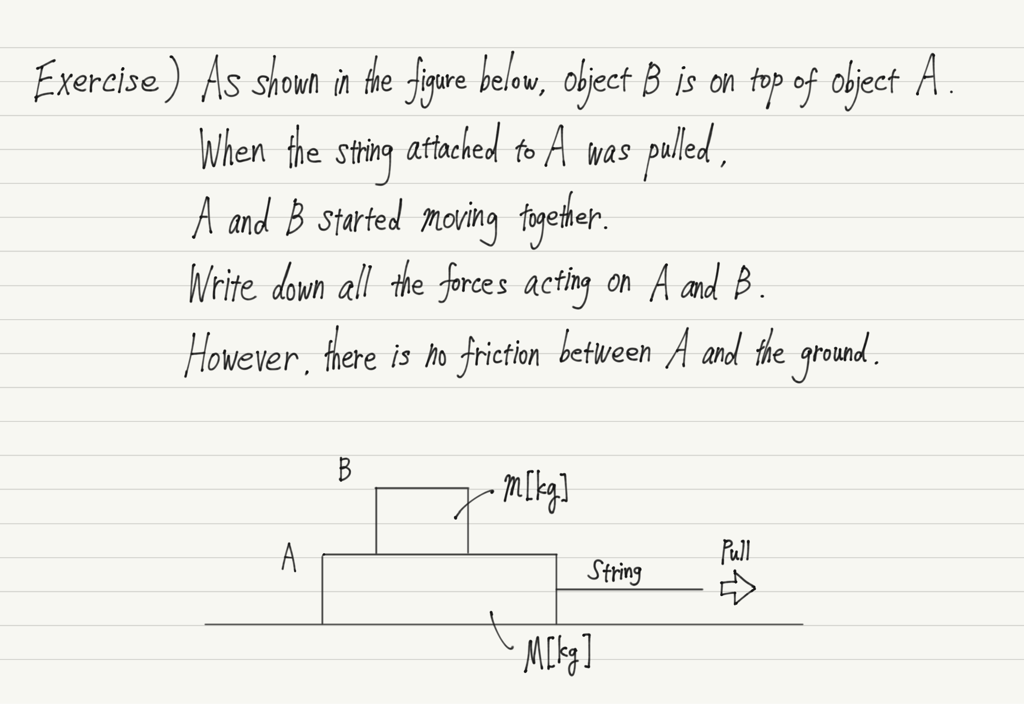Take what you’ve learned above and write your forces into it!

Let us explain. First, write gravitational force.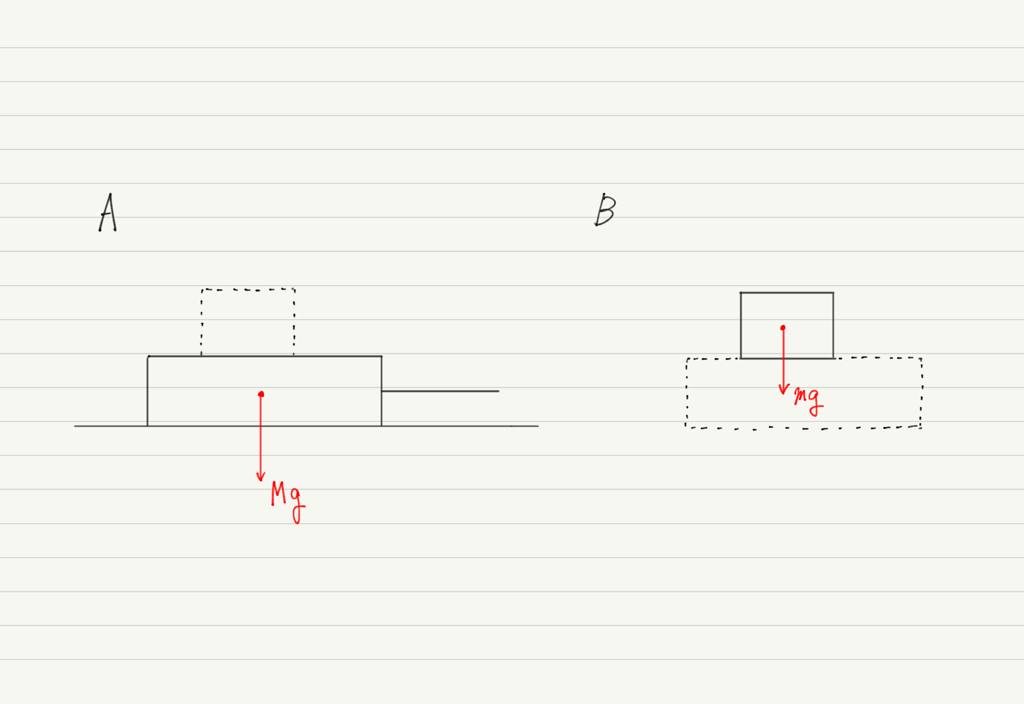Next, let us find the force received from the contact.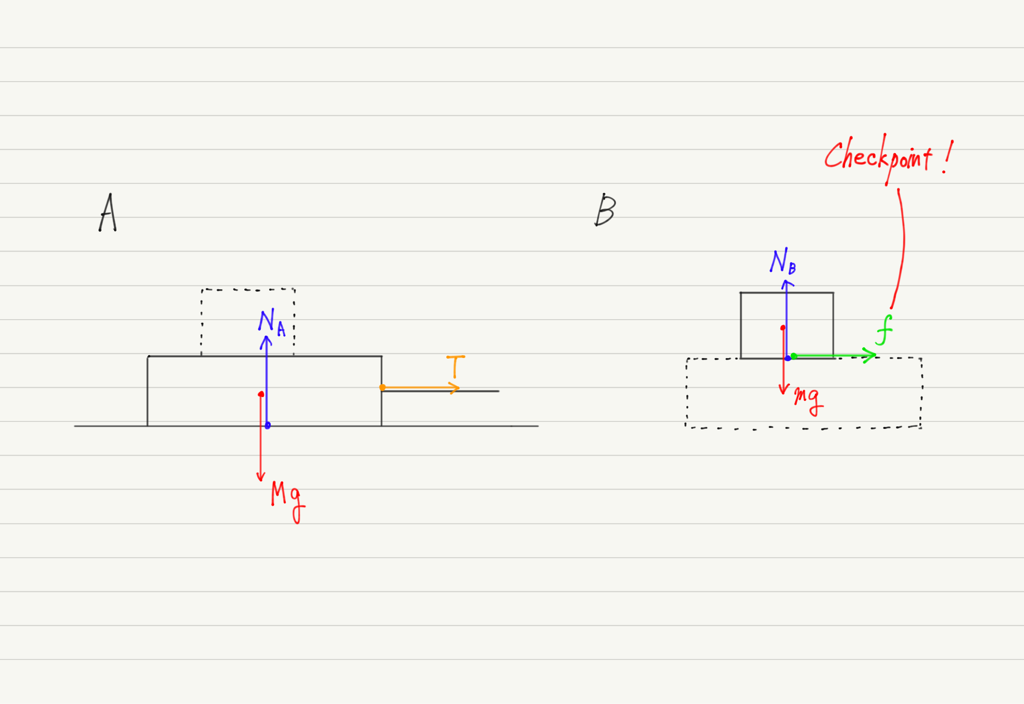I have included a checkpoint in the figure above, which is the presence of friction.

The problem statement never says there is a friction, but the sentence “A and B moved together” indicates that there is a friction between A and B.

The reason is that if there were no friction, only object A would be pulled out and object B would fall straight down.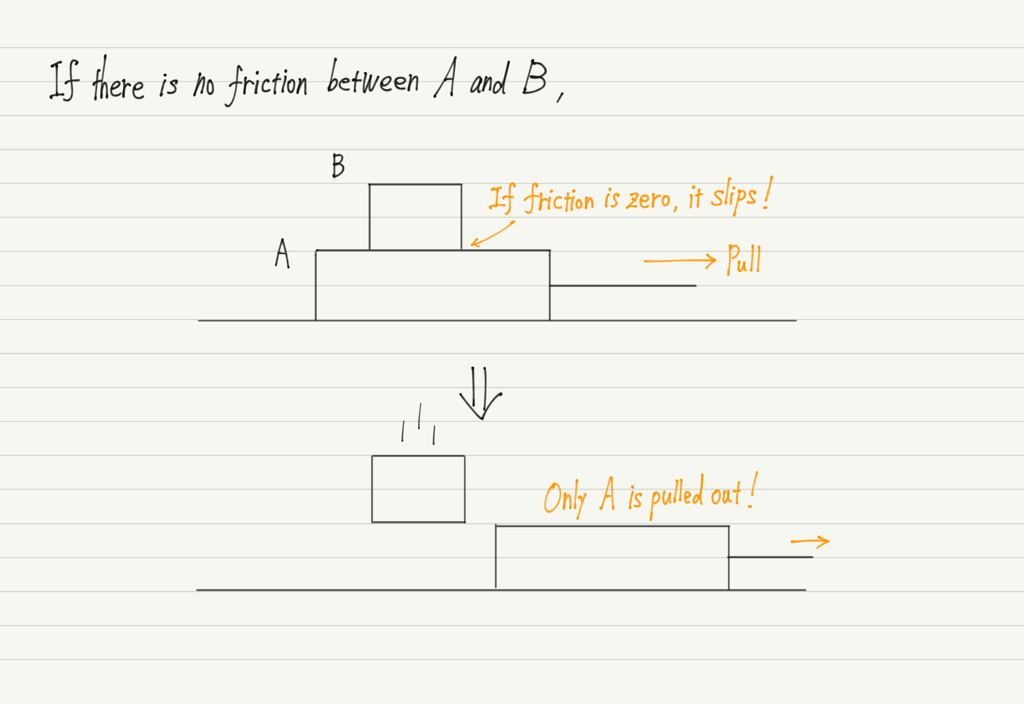In other words, a rightward force is absolutely necessary for a stationary B to start moving to the right.
Since B is only in contact with A, the force that moved B to the right can only be friction!

From the above, we can conclude that “a friction acts on B in the right direction.”

Now, since the explanation has become lengthy, I will reiterate the previous figure.Is that all?
Actually, there are two forces I haven’t written about yet!
Do you know what they are?

## Remember Action-Reaction

What is needed to get the final answer is the Law of action and reaction.

https://www.yukimura-physics.com/entry/dyn-f20

In the above figure, we haven’t written the reaction yet!
Add that, and this time it is complete.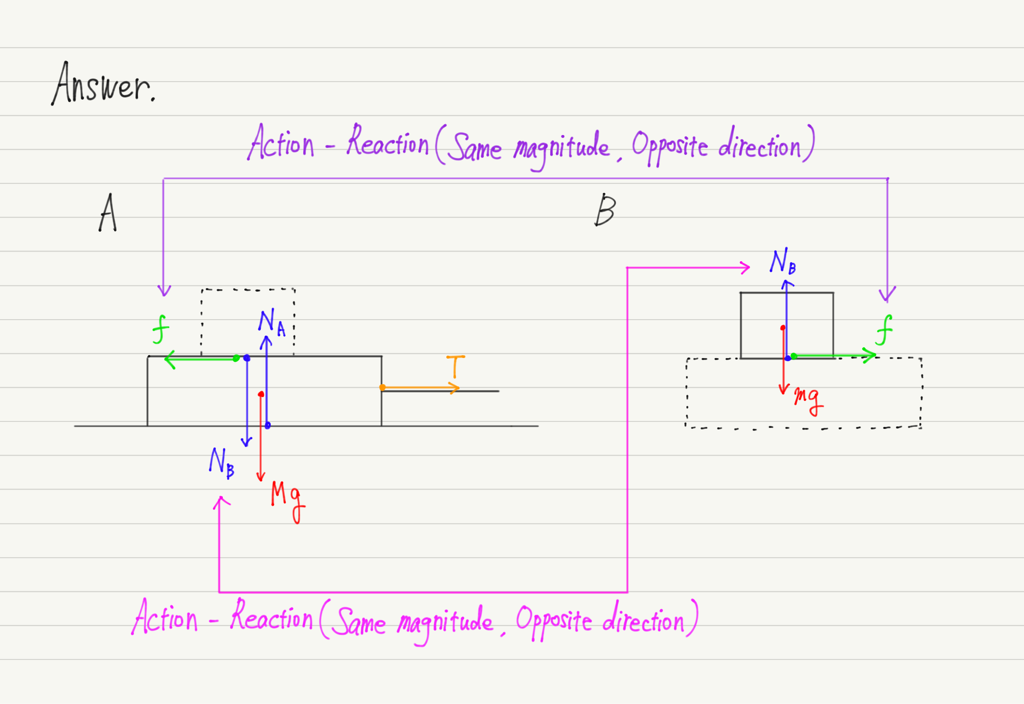Many people forget to write down the reaction, so be sure to check it at the end.

This lecture is only a preparation to formulate equations of motion.
Don’t be satisfied with just being able to write down the forces, but practice so that you can do the calculations as well!

error: Content is protected !!